Mrs. Tatum

•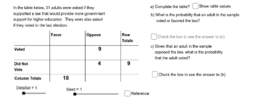Two-way Tables APS

Activity

Mrs. Tatum

•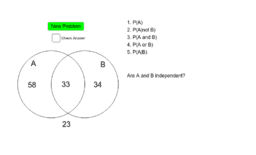Conditional Probabilities and Independence-APS

Activity

Mrs. Tatum

•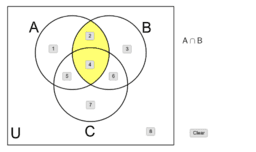Set Notation Unit 7 APS

Activity

Mrs. Tatum

•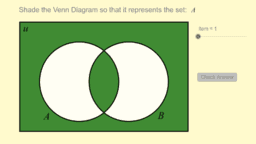Activity

Mrs. Tatum

•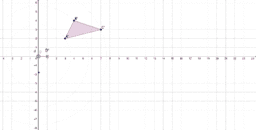Transformation Station Rotation APS

Activity

Mrs. Tatum

•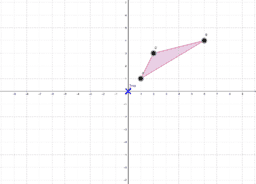Transformation Station Translation APS

Activity

Mrs. Tatum

•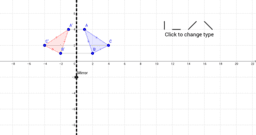Transformation Station Reflection APS

Activity

Mrs. Tatum

•Rigid Motions APS

Book

Mrs. Tatum

•Copying a Segment APS

Activity

Mrs. Tatum

•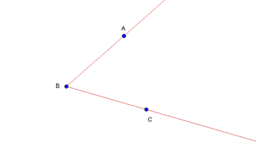Construction: Bisect an angle APS

Activity

Mrs. Tatum

•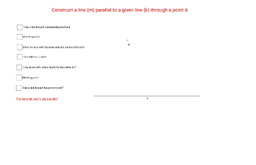Construct a line parallel to a line through a point - APS

Activity

Mrs. Tatum

•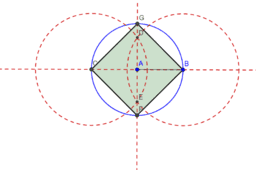Constructions: Square Inscribed in a Circle APS

Activity

Mrs. Tatum

•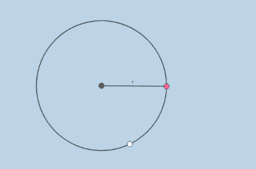Square Construction APS

Activity

Mrs. Tatum

•Construction: Regular Hexagon Inscribed in a Circle APS

Activity

Mrs. Tatum

•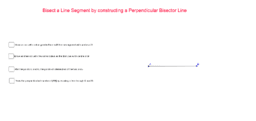Construction: Perpendicular Bisector of a Line Segment - APS

Activity

Mrs. Tatum

•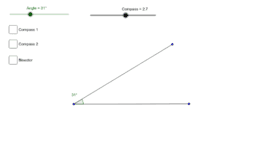Bisecting an Angle APS

Activity

Mrs. Tatum

•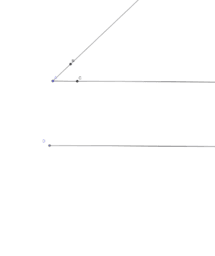Construction: Copy an Angle APS

Activity

Mrs. Tatum

•Bisecting a Segment 2 APS

Activity

Mrs. Tatum

•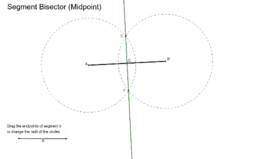Segment Bisector (Midpoint) APS

Activity

Mrs. Tatum

•Compass - Straightedge Construction - Copying a Segment APS

Activity

Mrs. Tatum

•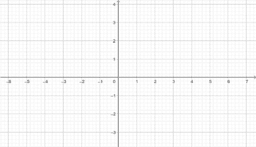Transformations in the coordinate plane APS

Book

Mrs. Tatum

•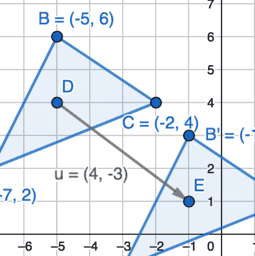Translating by Vector <a,b> APS

Activity

Mrs. Tatum

•Rotations in the Coordinate Plane APS

Activity

Mrs. Tatum

•Activity

Mrs. Tatum